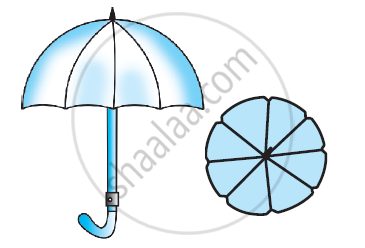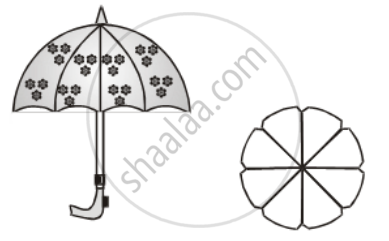# An umbrella has 8 ribs which are equally spaced. Assuming umbrella to be a flat circle of radius 45 cm. Find the area between the two consecutive ribs of the umbrella. - Mathematics

An umbrella has 8 ribs which are equally spaced. Assuming umbrella to be a flat circle of radius 45 cm. Find the area between the two consecutive ribs of the umbrella.#### Solution

Since ribs are equally spaced. Therefore, Angle made by two consecutive ribs at the centre = \frac { 360 }{ 8 } = 45ºThus,

Area between two consecutive ribs

= Area of a sector of a circle of radius 45 cm and sector angle 45º

={ \frac{45}{360}\times \frac{22}{7}\times 45\times 45}

= \frac { 1 }{ 8 } × \frac { 22 }{ 7 } × 45× 45 cm^2 = 795.53 cm^2

Concept: Areas of Sector and Segment of a Circle
Is there an error in this question or solution?
Chapter 12: Areas Related to Circles - Exercise 12.2 [Page 231]

#### APPEARS IN

NCERT Class 10 Maths
Chapter 12 Areas Related to Circles
Exercise 12.2 | Q 10 | Page 231
Share